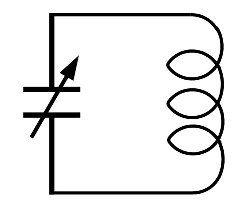top of page

## Capacitors:

Capacitor is also called as Condenser in electronic circuits.

The characteristics of a capacitor is measured in terms of Capacitance, which is the Quantity of Charge stored in a capacitor.

The units of Capacitance is Farads in S.I. system. One unit of Farad is very high for all practical purposes. So, microfarads , nanofarads , picofadas are used in the circuits.## Capacitance:

A capacitor contains two electrical conductos separated by a dielectric medium. Conductor of a capacitor may be a thin metallic foil or thin metal bead or an electrolyte. The nonconducting dielectric commonly used may be glass or ceramic or plastic film or paper or mica, air etc.

The capacity of Capacitor increases with decrease in the gap between the conductors and increase of the area of conductors## Fixed value Disc Capacitors:

Some disc capacitors are marked their capacitance in pico Farads (pF) in three digit coded form. The first two digits indicates value and third digit indicates number of zeroes followed. Refer examples shown below.

Fixed or Disc Capacitor does not have any polarity. The two pins may be connected to either positive and negative lines of the power supply or electronic signal. The capacitance value or code is printed on its surface. Maximum voltage it withstands are printed on some capacitors. The maximum value of these capacitors are 1 micro Farad and are available in lower fractions like nano Farads and Pico Farads## Fixed value Polarized Capacitors:

Polarized Capacitor contains negative terminal marked on its surface. i.e., a black band with negative (-) symbol. For new capacitors the negative terminal is kept shorter than positive terminal. These are mostly electrolytic (conducting material) capacitors.

The capacitance of these capacitors are normally starts from 1 micro Farad and available up to 10,000 micro Farads. The capacitance value and maximum voltage it withstands are printed on its surface.

Care should be taken while connecting a Polarized Capacitor to avoid damage. i.e. positive terminal of capacitor should be connected to positive supply line and negative terminal of capacitor should be connected to negative supply line only.## Variable value Capacitors:

Variable Capacitor can vary its capacitance by changing the conductor area by moving the conducting plates, normally by rotation. These capacitors normally have air as dielectric medium and its capacitance ranges in pico Farads.A tank circuit shown here, contains a capacitor and an inductor (coil) which generates frequency depending on the values of capacitance and inductance. A transistor is used to maintain the frequency with proper amplitude. These capacitors are mainly used in tank circuits, which generates oscillations and are used in radio (high frequency) circuits, for tuning the required frequency.## Capacitors in DC circuits:

Capacitors in DC circuits: Capacitors are used as temporary storage medium in DC (Direct Current) circuits. But, the charge across the conductors leaks through the dielectric medium after sometime. The leakage is high initially and slows down after some time.

A capacitor is used as filter in rectifier circuits to reduce the ripples of unregulated voltage, there by generate study voltage.

Initially a capacitor charges quickly then slows down the charging rate and matches to the source voltage (i.e., saturation level). Similarly, on discharge, the discharge rate is very high initially, then slows down and reaches to zero level (total discharge).## Capacitors in AC circuits:

Capacitor in AC circuits behaves differently than DC circuits. A change in voltage on conductor of one side of the capacitor effects on conductor of other side of the capacitor. The output signal is reversed to the input signal polarity. So, AC signal will be transferred in series using capacitor where as DC component will be blocked across the capacitor.

High frequencies and/or high capacitance makes easy passage of AC signal through the capacitor. The resistance offered in AC signal across a capacitor is called Impedence marked as Z. So, DC is considered as lowest frequency (almost zero frequency), therefore, the Impedence is highest (tends infinity) for DC signal.

## Capacitors in PARALLEL connection:

If multiple Capacitors are connected in parallel in electronic circuits, the resultant capacitance is equal to sum of each capacitance in the circuit.

So, the Resultant Capacitance is always more than any capacitance in parallel connection in the circuit.## Capacitors in SERIES connection:

If multiple capacitors are connected in series in a circuit, the resultant capacitance depends on the gap between the conductors of each capacitor. So, the Resultant gap is equal to sum of gaps between each capacitor in series. So, in terms of capacitance, inverse resultant capacitance is equal to sum of inverse capacitance of each capacitor in series. The Resultant Capacitance is always less than any capacitance value of any capacitor in the series connection in the circuit.

## SUPER CAPACITORS:

Super Capacitor is a capacitor with high-capacitance than electrolytic capacitor. It works as a low time storage battery. But, its storage voltage (normally available up to 5V) is very less comparative to normal capacitor.

The units used for Super Capacitors are in Farads and normally available in 1Farad or more.

bottom of page